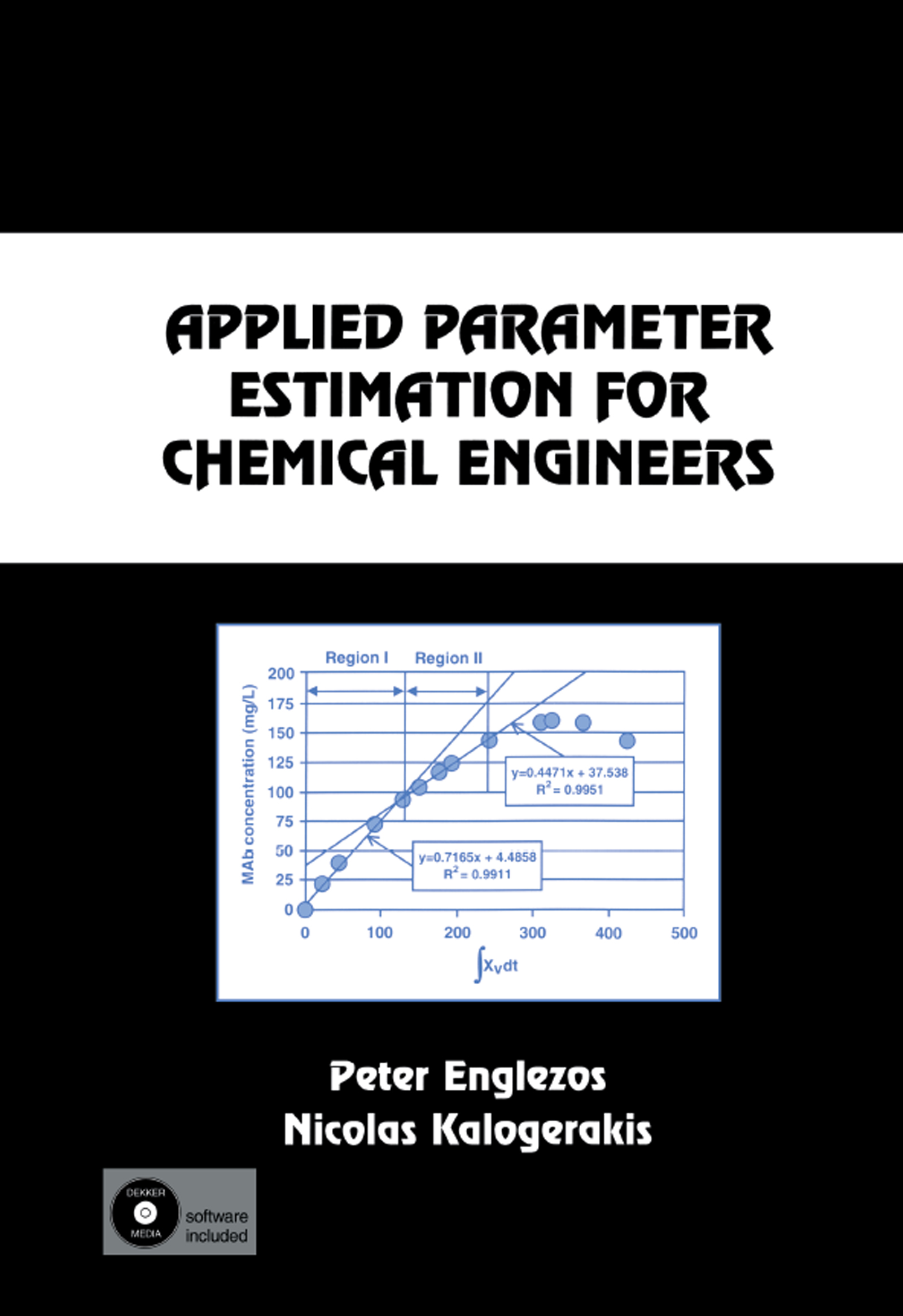# Applied Parameter Estimation for Chemical Engineers

## 1st Edition

CRC Press

460 pages

Paperback: 9780367398026
pub: 2019-09-05
SAVE ~\$14.99
\$74.95
\$59.96
x
Hardback: 9780824795610
pub: 2000-10-12
SAVE ~\$60.00
\$300.00
\$240.00
x

FREE Standard Shipping!

### Description

This book determines adjustable parameters in mathematical models that describe steady state or dynamic systems, presenting the most important optimization methods used for parameter estimation. It focuses on the Gauss-Newton method and its modifications for systems and processes represented by algebraic or differential equation models.

Formulation of the parameter estimation problem; computation of parameters in linear models-linear regression; Gauss-Newton method for algebraic models; other nonlinear regression methods for algebraic models; Gauss-Newton method for ordinary differential equation (ODE) models; shortcut estimation methods for ODE models; practical guidelines for algorithm implementation; constrained parameter estimation; Gauss-Newton method for partial differential equation (PDE) models; statistical inferences; design of experiments; recursive parameter estimation; parameter estimation in nonlinear thermodynamic models - cubic equation of state; parameter estimation in nonlinear thermodynamic models - activity coefficients; parameter estimation in chemical reaction kinetic models; parameter estimation in biochemical engineering models; parameter estimation in petroleum engineering. Appendices: the Trebble-Bishnoi equation of state; derivation of the fugacity expression; listings of computer programs; contents of accompanying CD; computer program for example16.1.2; computer program for example 16.3.2.